Chapter 7. Energy and Chemistry

# Energy

Learning Objectives

1. Define energy.
2. Know the units of energy.
3. Understand the law of conservation of energy.

is the ability to do work. Think about it: when you have a lot of energy, you can do a lot of work; but if you’re low on energy, you don’t want to do much work. Work (w) itself is defined as a force (F) operating over a distance (Δx):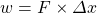In SI, force has units of newtons (N), while distance has units of metres. Therefore, work has units of N⋅m (Newton-metres). This compound unit is redefined as a (J):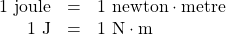Because energy is the ability to do work, energy is also measured in joules. This is the primary unit of energy we will use here.

How much is 1 J? It is enough to warm up about one-fourth of a gram of water by 1°C. It takes about 12,000 J to warm a cup of coffee from room temperature to 50°C. So a joule is not a lot of energy. It is not uncommon to measure energies in thousands of joules, so the kilojoule (kJ) is a common unit of energy, with 1 kJ equal to 1,000 J.

An older — but still common — unit of energy is the . The calorie (cal) was originally defined in terms of warming up a given quantity of water. The modern definition of calorie equates it to joules: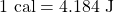One area where the calorie is used is in nutrition. Energy contents of foods are often expressed in calories. However, the calorie unit used for foods is actually the kilocalorie (kcal). Most foods indicate this by spelling the word with a capital C — Calorie. Figure 7.1 “Calories on Food Labels” shows one example.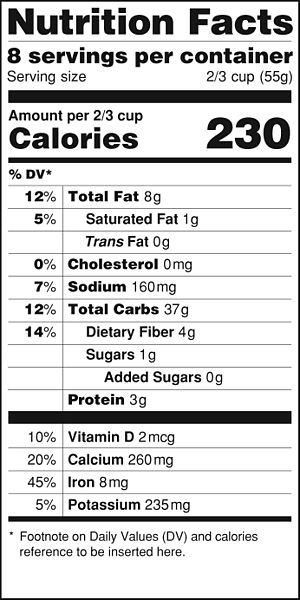Figure 7.1 “Calories on Food Labels.” This label expresses the energy content of the food, but in Calories (which are actually kilocalories).

Example 7.5

# Problem

The label in Figure 7.1 “Calories on Food Labels” states that the serving has 38 Cal. How many joules is this?

## Solution

We recognize that with a capital C, the Calories unit is actually kilocalories. To determine the number of joules, we convert first from kilocalories to calories (using the definition of the kilo- prefix) and then from calories to joules (using the relationship between calories and joules). So: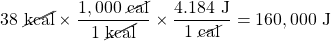# Test Yourself

A serving of breakfast cereal usually has 110 Cal. How many joules of energy is this?

460,000 J

In the study of energy, we use the term  to describe the part of the universe under study: a beaker, a flask, or a container whose contents are being observed and measured. An  is a system that does not allow a transfer of energy or matter into or out of the system. A good approximation of an isolated system is a closed, insulated thermos-type bottle. The fact that the thermos-type bottle is closed keeps matter from moving in or out, and the fact that it is insulated keeps energy from moving in or out.

One of the fundamental ideas about the total energy of an isolated system is that is does not increase or decrease. When this happens to a quantity, we say that the quantity is conserved. The statement that the total energy of an isolated system does not change is called the . As a scientific law, this concept occupies the highest level of understanding we have about the natural universe.

Key Takeaways

• Energy is the ability to do work and uses the unit joule.
• The law of conservation of energy states that the total energy of an isolated system does not increase or decrease.

Exercises

# Questions

1. Define energy. How is work related to energy?
2. Give two units of energy and indicate which one is preferred.
3. Express the quantity of 422 J in calories.
4. Express the quantity of 3.225 kJ in calories.
5. Express the quantity 55.69 cal in joules.
6. Express the quantity 965.33 kcal in joules.
7. How does a Calorie differ from a calorie?
8. Express the quantity 965.33 Cal in joules.
9. What is the law of conservation of energy?
10. What does the word conserved mean as applied to the law of conservation of energy?

1. Energy is the ability to do work. Work is a form of energy.
1. 101 cal
1. 233.0 J
1. A Calorie is actually a kilocalorie, or 1,000 calories.
1. The total energy of an isolated system does not increase or decrease.